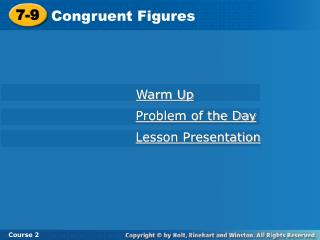# 7-9 - PowerPoint PPT PresentationDownload Presentation7-9

7-9Download Presentation## 7-9

- - - - - - - - - - - - - - - - - - - - - - - - - - - E N D - - - - - - - - - - - - - - - - - - - - - - - - - - -
##### Presentation Transcript

1. 7-9 Congruent Figures Course 2 Warm Up Problem of the Day Lesson Presentation

2. 7-9 Congruent Figures Course 2 Warm Up Find the measure of the third angle in the triangle, given two angle measures. 1.58°, 104° 2. 32°, 63° 3. 90°, 38° 4. 42°, 88° 18° 85° 52° 50°

3. 7-9 Congruent Figures C F D E A B Course 2 Problem of the Day Draw triangles ABC and DEF so that a.AB = DE and BC = EF b. angle A is congruent to angle D. c. the triangles are not congruent.

4. 7-9 Congruent Figures Course 2 Learn to identify congruent figures and to use congruence to solve problems.

5. 7-9 Congruent Figures Course 2 Insert Lesson Title Here Vocabulary Side-Side-Side Rule

6. 7-9 Congruent Figures Course 2 Which center circle do you think is bigger? In spite of appearances, the two center circles are congruent. Their apparent differences are optical illusions. One way to determine whether figures are congruent is to see if one figure will fit exactly over the other one. Look at the two patterns.

7. 7-9 Congruent Figures Course 2 Additional Example 1A: Identifying Congruent Figures in the Real World Identify any congruent figures. A. The sides of the octagons are not congruent. Each side of the outer figure is larger than each side of the inner figure.

8. 7-9 Congruent Figures Course 2 Additional Example 1B: Identifying Congruent Figures in the Real World Identify any congruent figures. B. The buttons labeled 1, 4, 7, and * are congruent. The buttons labeled 2, 5, 8, and 0 are also congruent. Also the buttons labeled 3, 6, 9, and # are congruent.

9. 7-9 Congruent Figures Course 2 If all of the corresponding sides and angles of two polygons are congruent, then the polygons are congruent. For triangles in particular, the corresponding angles will always be congruent if the corresponding sides are congruent. This is called the Side-Side-Side Rule. Because of this rule, when determining whether triangles are congruent, you only need to determine whether the sides are congruent.

10. 7-9 Congruent Figures Course 2 Additional Example 2: Identifying Congruent Triangles Determine whether the triangles are congruent. Q C 4 cm 4 cm AB = 4 cm BC = 4 cm AC = 6 cm PQ = 4 cm PR = 4 cm RQ = 5 cm B P 5 cm 6 cm 4 cm 4 cm R A The triangles are not congruent. Although two sides in one triangle are congruent to two sides in the other, the third sides are not congruent.

11. 7-9 Congruent Figures By the Side-Side-Side Rule, ABC is congruent to DEF, or ABCDEF. If you flip one triangle, it will fit exactly over the other. Reading Math The notation ABC is read “triangle ABC.” Course 2 Try This: Example 2 Determine whether the triangles are congruent. AC = 8 m AB = 6 m BC = 10 m DF = 8 m DE = 6 m EF = 10 m B E 10 m 10 m 6 m 6 m A C D F 8 m 8 m

12. 7-9 Congruent Figures 120 m 120 m 70 m 70 m 70 m 70 m 120 m 120 m Course 2 For polygons with more than three sides, it is not enough to compare the measures of their sides. For example, the corresponding sides of the figures below are congruent, but the figures are not congruent. 145° 35° 35° 145° If you know that two figures are congruent, you can find the missing measures in the figures.

13. 7-9 Congruent Figures Course 2 Additional Example 3A: Using Congruence to Find Missing Measures Determine the missing measures in the set of congruent polygons. The corresponding sides are congruent. The missing side measure is 28 mm.

14. 7-9 Congruent Figures Course 2 Additional Example 3B: Using Congruence to Find Missing Measures Determine the missing measure in the set of congruent polygons. The corresponding angles are congruent. The missing angle measure is 88°.

15. 7-9 Congruent Figures Course 2 Insert Lesson Title Here Try This: Example 3A Determine the missing measure in the set of congruent polygons. 135° 5 ft The corresponding sides are congruent. 120° 6 ft 45° 60° 8 ft The missing side measure is 4 ft. 8 ft 60° 45° 6 ft 135° 5 ft 120° 4 ft

16. 7-9 Congruent Figures Course 2 Insert Lesson Title Here Try This: Example 3B Determine the missing measure in the set of congruent polygons. 6 ft 5 ft 130° 110° 8 ft The corresponding angles are congruent. 120° 80° 4 ft 100° 10 ft The missing angle measure is 130°. 10 ft 100° 4 ft 80° 120° 8 ft 110° ? 5 ft 6 ft

17. 7-9 Congruent Figures D A 5 cm 5 cm 7 cm 8 cm E 8 cm B 8 cm C F Course 2 Insert Lesson Title Here Lesson Quiz no 1. Are the triangles congruent? 2. Determine the missing measures for the set of congruent polygons. 11 70° 70° 110° c 6 6 6 b 70° 70° 110° 110° 18 a a =11, b =6, c = 110°